﻿ 绳驱超冗余空间柔性机械臂遥操作系统设计与实验研究
 机器人2022, Vol. 44Issue (1): 9-180

ZHU Xiaojun, WANG Xueqian, MA Yunxuan, LIANG Bin, SUN Hanxu. Design and Experimental Study on the Teleoperation System for Cable-driven and Hyper-redundant Space Flexible Manipulator[J]. ROBOT, 2022, 44(1): 9-18.1. 北京邮电大学自动化学院, 北京 100876;
2. 清华大学深圳国际研究生院, 广东 深圳 518055;
3. 清华大学自动化系, 北京 100084

Design and Experimental Study on the Teleoperation System for Cable-driven and Hyper-redundant Space Flexible Manipulator
ZHU Xiaojun1 , WANG Xueqian2, MA Yunxuan2 , LIANG Bin3 , SUN Hanxu1
1. School of Automation, Beijing University of Posts and Telecommunications, Beijing 100876, China;
2. Tsinghua Shenzhen International Graduate School, Shenzhen 518055, China;
3. Department of Automation, Tsinghua University, Beijing 100084, China
Abstract: For the motion characteristics of cable-driven and hyper-redundant flexible manipulator and the requirements of on orbit teleoperation tasks such as rapid movement in a large work space, obstacle avoidance, narrow space operation and fine operation under collision conditions, a human-machine interaction system for teleoperation based on the combination of manual controller and virtual reality (VR) handle is designed and developed, which can adapt to a variety of working conditions. Based on the analysis on working conditions such as narrow space operation and obstacle avoidance, a teleoperation method is proposed based on the DOF (degree of freedom) dynamic combination and the synchronous planning of the end and the arm configuration. Finally, a typical experiment of crossing through the slit between satellite solar panels is carried out. The experiment verifies the engineering practicability of the teleoperation system and the feasibility of the teleoperation motion planning method. It shows that the combination of a variety of human-machine interaction modes of teleoperation system and the corresponding motion planning method enable the operator to participate in the teleoperation system in a more intuitive and natural way, and improve the safety and operation performance of the operator to complete complex teleoperation tasks effectively.
Keywords: space flexible manipulator    cable-driven    teleoperation    human-machine interaction    motion planning

1 引言（Introduction）

2 超冗余空间柔性机械臂遥操作系统设计（The teleoperation system design for hyper-redundant space flexible manipulator）

2.1 超冗余柔性机械臂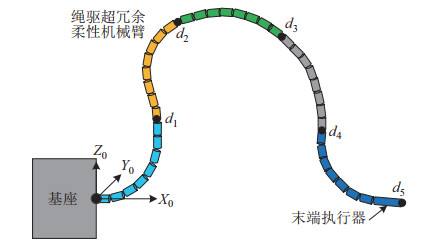图 1 绳驱超冗余柔性机械臂示意图 Fig.1 The schematic of cable-driven and hyper-redundant flexible manipulator表 1 单个柔性模块关节参数 Tab. 1 Joint parameters of a single flexible module
2.2 超冗余空间柔性机械臂遥操作人机交互系统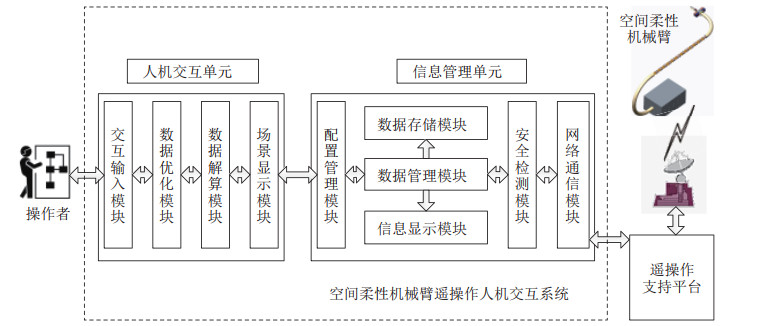图 2 遥操作系统组成图 Fig.2 The composition diagram of the teleoperation system

(1) 人机交互单元在遥操作系统中主要承担将操作者的意图转化为柔性机械臂控制指令的功能，由交互输入模块、数据优化模块、数据解算模块、场景显示模块组成。一方面操作者通过人机交互设备产生自然高效的控制指令，另一方面，人机交互单元给操作者提供充分的远端环境临场感信息。

(2) 信息管理单元在遥操作系统中主要承担数据的接收、转发、存储，指令的安全检测，系统配置管理等功能，由配置管理模块、数据存储模块、数据管理模块、信息显示模块、安全检测模块、网络通信模块组成。信息管理单元是遥操作系统与外界的连接中枢。

2.3 人机交互方式表 2 人机交互设备 Tab. 2 Human-machine interaction equipments
2.3.1 力反馈手控器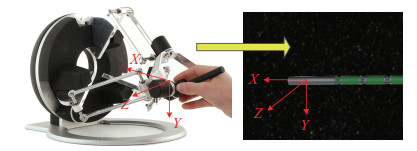图 3 手控器控制示意图 Fig.3 The schematic of the hand controller
2.3.2 VR手柄

VR手柄的优点是人机交互直观自然、操作空间大、速度快，属于非接触式的人机交互设备，整个操作方式是非浸入式的，对操作员的干扰大大减小，应用于需要避障操作或大范围工作空间运动的工作场景[13-14]。操作员使用2个手柄，一个手柄控制柔性机械臂末端位姿，另一个手柄控制柔性机械臂中间臂形控制点，如图 4所示。对于长度达4 m的柔性机械臂只需一个操作者即可同时控制柔性臂的臂形与末端。VR设备采用Oculus VR公司推出的Oculus Rift头戴式虚拟现实设备，包括Oculus CV1眼镜、VR Touch手柄和定位器。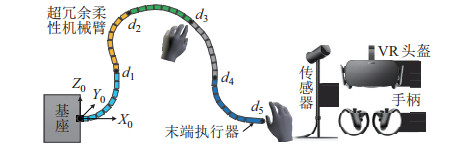图 4 VR手柄控制柔性机械臂示意图 Fig.4 The schematic of flexible manipulator controlled by VR handle
3 超冗余柔性机械臂的运动规划算法（The motion planning algorithm of hyper-redundant flexible manipulator） 3.1 运动学建模

 \begin{align} \begin{cases} {{\mathit{\boldsymbol{v}}}}_{m, 1} ={{\mathit{\boldsymbol{e}}}}_{m, 1} \times {{\mathit{\boldsymbol{r}}}}_{m, 1} +{{\mathit{\boldsymbol{e}}}}_{m, 4} \times {{\mathit{\boldsymbol{r}}}}_{m, 4} +{{\mathit{\boldsymbol{e}}}}_{m, 5} \times {{\mathit{\boldsymbol{r}}}}_{m, 5} +\\ \qquad\; \; \; {{\mathit{\boldsymbol{e}}}}_{m, 8} \!\times\! {{\mathit{\boldsymbol{r}}}}_{m, 8} \!+\! {{\mathit{\boldsymbol{e}}}}_{m, 9} \!\times\! {{\mathit{\boldsymbol{r}}}}_{m, 9} \!+\! {{\mathit{\boldsymbol{e}}}}_{m, 12} \!\times\! {{\mathit{\boldsymbol{r}}}}_{m, 12}+\\ \qquad\; \; \; {{\mathit{\boldsymbol{e}}}}_{m, 13} \times {{\mathit{\boldsymbol{r}}}}_{m, 13} +{{\mathit{\boldsymbol{e}}}}_{m, 16} \times {{\mathit{\boldsymbol{r}}}}_{m, 16}\\ {{\mathit{\boldsymbol{v}}}}_{m, 2} ={{\mathit{\boldsymbol{e}}}}_{m, 2} \!\times\! {{\mathit{\boldsymbol{r}}}}_{m, 2} \!+\! {{\mathit{\boldsymbol{e}}}}_{m, 3} \!\times\! {{\mathit{\boldsymbol{r}}}}_{m, 3} \!+\! {{\mathit{\boldsymbol{e}}}}_{m, 6} \!\times\! {{\mathit{\boldsymbol{r}}}}_{m, 6} +\\ \qquad\; \; \; {{\mathit{\boldsymbol{e}}}}_{m, 7} \!\times\! {{\mathit{\boldsymbol{r}}}}_{m, 7} \!+\! {{\mathit{\boldsymbol{e}}}}_{m, 10} \!\times\! {{\mathit{\boldsymbol{r}}}}_{m, 10} \!+\! {{\mathit{\boldsymbol{e}}}}_{m, 11} \!\times\! {{\mathit{\boldsymbol{r}}}}_{m, 11} + \\ \qquad\; \; \; {{\mathit{\boldsymbol{e}}}}_{m, 14} \times {{\mathit{\boldsymbol{r}}}}_{m, 14} +{{\mathit{\boldsymbol{e}}}}_{m, 15} \times {{\mathit{\boldsymbol{r}}}}_{m, 15} \end{cases}\!\!\!\!\!\! \end{align} (6)

 \begin{align} \mathit{\boldsymbol{w}}_{m} =\;&\mathit{\boldsymbol{e}}_{m, 1} \cdot \dot{\theta}_{2m-1} +\mathit{\boldsymbol{e}}_{m, 2} \cdot \dot{\theta}_{\rm{2m}} +\cdots +\mathit{\boldsymbol{e}}_{m, 15} \cdot \dot{\theta}_{\rm{2m}} +\\ &\mathit{\boldsymbol{e}}_{m, 16} \cdot \dot{\theta}_{2m-1} \\ =\;&[{\mathit{\boldsymbol{w}}_{m, 1}}\; \; {\mathit{\boldsymbol{w}}_{m, 2}}] \begin{bmatrix} {\dot{\theta}_{2m-1}} \\[-3pt] {\dot{\theta}_{2m}} \end{bmatrix} \end{align} (7)

\mathit{\boldsymbol{w}}_{m, k} k=1, 2 ）代表关节段 m 中相同方向轴 k 的单位角速度对末端角速度的影响，可以具体表示为  \begin{align} \begin{cases} \mathit{\boldsymbol{w}}_{m, 1} =\mathit{\boldsymbol{e}}_{m, 1} +\mathit{\boldsymbol{e}}_{m, 4} +\mathit{\boldsymbol{e}}_{m, 5} +\mathit{\boldsymbol{e}}_{m, 8}+\\ \phantom{\mathit{\boldsymbol{w}}_{m, 1} =\; } \mathit{\boldsymbol{e}}_{m, 9} +\mathit{\boldsymbol{e}}_{m, 12} +\mathit{\boldsymbol{e}}_{m, 13} +\mathit{\boldsymbol{e}}_{m, 16} \\ \mathit{\boldsymbol{w}}_{m, 2} =\mathit{\boldsymbol{e}}_{m, 2} +\mathit{\boldsymbol{e}}_{m, 3} +\mathit{\boldsymbol{e}}_{m, 6} +\mathit{\boldsymbol{e}}_{m, 7}+\\ \phantom{\mathit{\boldsymbol{w}}_{m, 1} =\; } \mathit{\boldsymbol{e}}_{m, 10} +\mathit{\boldsymbol{e}}_{m, 11} +\mathit{\boldsymbol{e}}_{m, 14} +\mathit{\boldsymbol{e}}_{m, 15} \end{cases} \end{align} (8) 进一步地可以得到：  \begin{align} \mathit{\boldsymbol{J}}_{m} \mathit{\boldsymbol{\dot{\theta}}}_{m} =\begin{bmatrix} {\mathit{\boldsymbol{v}}_{m}} \\[-3pt] {\mathit{\boldsymbol{w}}_{m}} \end{bmatrix}\mathit{\boldsymbol{\dot{\theta}}}_{m} =\begin{bmatrix} {\mathit{\boldsymbol{v}}_{m, 1}} & {\mathit{\boldsymbol{v}}_{m, 2}} \\[-3pt] {\mathit{\boldsymbol{w}}_{m, 1}} & {\mathit{\boldsymbol{w}}_{m, 2}} \end{bmatrix}\begin{bmatrix} {\dot{\theta}_{2m-1}} \\[-3pt] {\dot{\theta}_{2m}} \end{bmatrix} \end{align} (9) 再结合式(3) 可以得到简化后的雅可比矩阵：  \begin{align} \mathit{\boldsymbol{J}}_{m} =\begin{bmatrix} {\mathit{\boldsymbol{v}}_{m, 1}} & {\mathit{\boldsymbol{v}}_{m, 2}} \\[-3pt] {\mathit{\boldsymbol{w}}_{m, 1}} & {\mathit{\boldsymbol{w}}_{m, 2}} \end{bmatrix} \end{align} (10) 整个柔性机械臂的雅可比矩阵的推导与单个关节段的类似，最后得到末端线速度和角速度与各个轴的关系：  \begin{align} \mathit{\boldsymbol{J}}\mathit{\boldsymbol{\dot{\theta}}} =\begin{bmatrix} {\mathit{\boldsymbol{v}}_{1, 1}} & {\mathit{\boldsymbol{v}}_{1, 2}} & \cdots & {\mathit{\boldsymbol{v}}_{5, 2}} \\[-3pt] {\mathit{\boldsymbol{w}}_{1, 1}} & {\mathit{\boldsymbol{w}}_{1, 2}} & \cdots & {\mathit{\boldsymbol{w}}_{5, 2}} \end{bmatrix} [{\dot{\theta}_{1}} \; \; {\dot{\theta}_{2}} \; \; \cdots \; \; {\dot{\theta}_{10}} ]^{\rm T} \end{align} (11) 即超冗余柔性机械臂的雅可比矩阵为  \begin{align} \mathit{\boldsymbol{J}} =\begin{bmatrix} {\mathit{\boldsymbol{v}}_{1, 1}} & {\mathit{\boldsymbol{v}}_{1, 2}} & \cdots & {\mathit{\boldsymbol{v}}_{5, 2}} \\ {\mathit{\boldsymbol{w}}_{1, 1}} & {\mathit{\boldsymbol{w}}_{1, 2}} & \cdots & {\mathit{\boldsymbol{w}}_{5, 2}} \end{bmatrix}^{6\times 10} \end{align} (12) 3.2 基于自由度动态组合的柔性机械臂规划方法 针对狭小空间作业的需求，提出基于自由度动态组合的规划方法。柔性机械臂在维修太阳帆板压紧杆的任务中，其最为困难的地方在于实现臂本体在帆板狭缝中穿越。基于此，柔性臂处于帆板狭缝之间的部分需要进行平面规划，并保持构形不变，而处于帆板外面的部分则需要进行空间规划。因此，可以将柔性机械臂划分为帆板外 S_{1} 和帆板内 S_{2} 两大部分，如图 6所示。对于柔性臂帆板内大段 S_{2} ，可以通过给定的末端点的位置，利用几何求解的方法求解其根部的可能范围。剩余的自由度 N 由逆运动学算法计算确定。由于在迭代计算过程中柔性机械臂参与求逆解的自由度数目是动态变化的，由此提出了基于自由度动态组合法的空间逆解规划方法，完成柔性机械臂穿越帆板狭小空间时的轨迹规划。根据帆板外 S_{1} 和帆板内 S_{2} 两大部分的规划需求，两大部分的自由度动态组合的方式有：10DOF + 0DOF、9DOF + 1DOF、8DOF + 2DOF、……。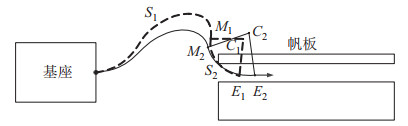图 6 自由度动态组合规划方法示意图 Fig.6 The planning method based on DOF dynamic combination  \begin{align} {}^{\rm 0}{{\mathit{\boldsymbol{T}}}}_{\rm M} ={}^{\rm 0}{{\mathit{\boldsymbol{T}}}}_{\rm end} {{\mathit{\boldsymbol{f}}}}_{S_{2}}^{-1} (\mathit{\boldsymbol{\theta}}_{S_{2}}) \end{align} (13) 式(13) 中解出了柔性臂段 S_{2} 中的俯仰自由度，剩下的自由度则结合柔性机械臂逆运动学算法，在每次逆解计算过程中，其实际迭代用到的关节角个数为10、9、8个。 根据自由度的变化情况，柔性机械臂穿越帆板狭缝的规划过程可以分为3个子过程，帆板外采用10DOF + 0DOF方式进行规划，第5段进入狭缝时采用9DOF + 1DOF方式进行规划，第4、5段进入狭缝时采用8DOF + 2DOF方式进行规划，仿真过程如图 7所示。由图 7可知，采用此方法可以有效地完成帆板狭缝穿越任务。在帆板狭缝穿越任务中，由于帆板形成的空间是一个平面，将末1段或末2段的俯仰角度设置为固定值0 ^{\circ} ，其他工作场景 S_{2} 段俯仰角度根据不同任务需求进行配置计算。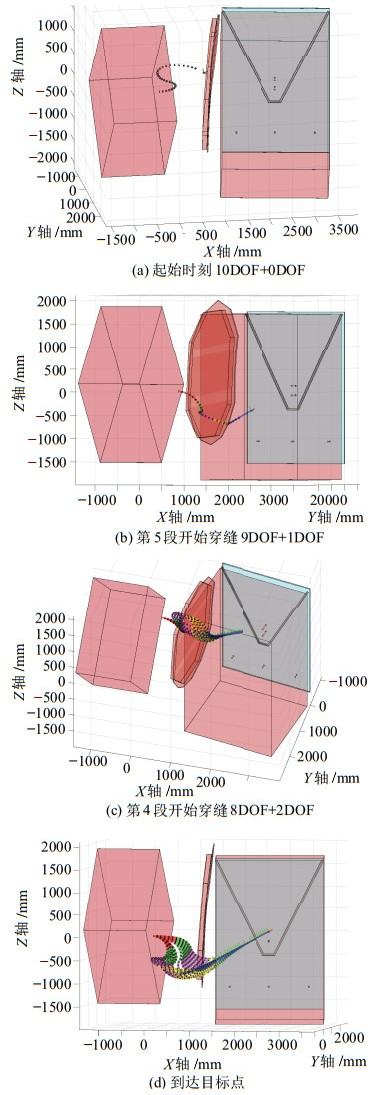图 7 自由度动态组合规划仿真 Fig.7 Simulation of the planning method based on DOF dynamic combination 3.3 末端—臂形同步规划方法 当超冗余柔性机械臂需要完成避障规划时，需要对末端位姿和臂形进行整体规划。针对这一问题，提出将超冗余柔性机械臂段与段之间的控制点作为控制对象来调整机械臂臂形的方法，该方法在满足末端位姿到达的前提下，通过优化超冗余柔性机械臂所有自由度的方式，使中间臂形逼近控制点要求的整体臂形，因此，该方法满足了“整臂臂形—末端轨迹”同步规划的需求。 在末端轨迹与期望轨迹一致的基础上，在空间设置 j 个控制目标点，在超冗余柔性机械臂设定 j 个被控制点。根据机械臂的特点将被控制点设定为机械臂上关节段与段之间的节点 N_{j} {j=1, 2, 3, 4}），被控制点与相应控制目标点$C_{j} $${j=1, 2, 3, 4} ）之间的距离 d_{j} =| {\overrightarrow {N_{j} C_{j}}} |$$ {j=1, 2, 3, 4}$）则为待优化目标，如图 8所示。当任意欧氏距离大于对应安全性阈值时，通过基于梯度投影的全局优化法使得欧氏距离$d_{i}$逼近0，从而得到超冗余柔性机械臂在空间的期望构形。如果任意欧氏距离$d_{i}$为0，则超冗余机械臂的构形与控制点设定的期望构形完全一致。如果任意欧氏距离$d_{j}$不为0，则通过优化超冗余柔性机械臂的整臂自由度，使得距离$d_{i}$的加权和$\sum\limits_{i=1}^4 {\lambda_{j} d_{j}}$最小（$\lambda_{j}$为权重系数），即柔性机械臂的整臂构形与控制点所设定构形的一致性达到最优化状态，实现末端—臂形同步规划目标。针对文中柔性机械臂任务特点，主要优先调整$d_{3}$点位置来控制臂形。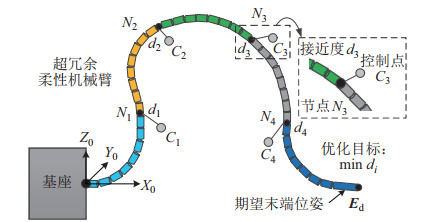图 8 末端—臂形同步规划示意图 Fig.8 Synchronous planning of the end and the arm configuration
4 超冗余空间柔性机械臂遥操作系统研制与实验（Development and experiment of the teleoperation system for a hyper-redundant space flexible manipulator） 4.1 遥操作人机交互系统的实现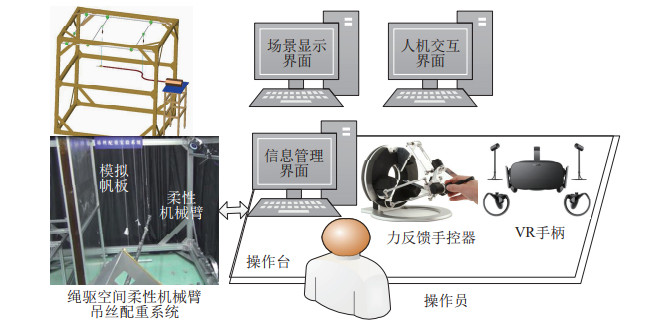图 9 遥操作人机交互系统布局图 Fig.9 The schematic of teleoperation human-machine interaction system
4.2 柔性机械臂遥操作实验

4.2.1 实验条件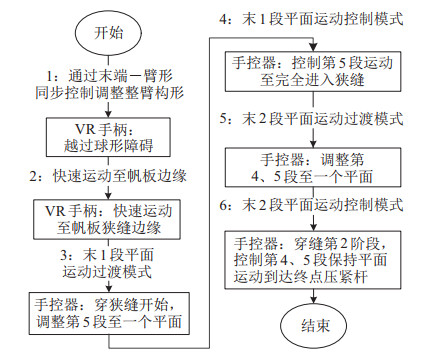图 10 实验步骤流程图 Fig.10 Flow chart of experimental steps
4.2.2 实验结果与分析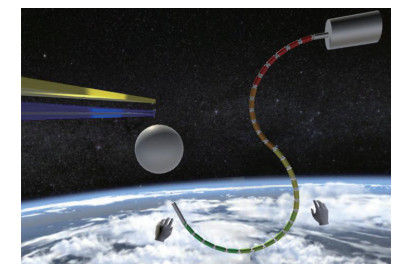图 11 柔性机械臂穿越帆板的准备臂形 Fig.11 The prepared arm configuration of flexible manipulator crossing through the solar panels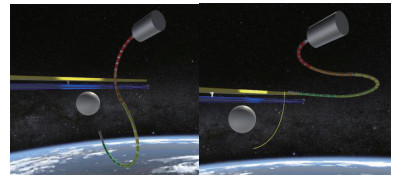图 12 机械臂通过末端—臂形同步控制运动至帆板边缘 Fig.12 The manipulator moving to the edge of the solar panel by synchronous control of the end and the arm configuration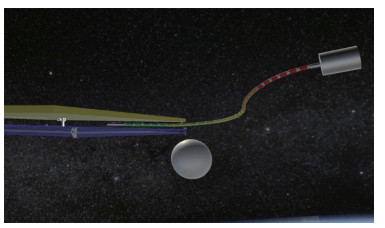图 13 第5段保持平面运动进入狭缝 Fig.13 The 5th segment keeps the plane moving into the slit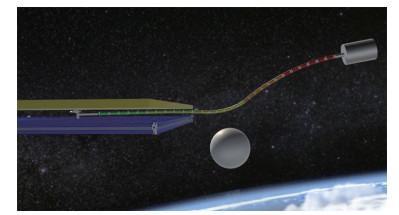图 14 第4、5段保持平面进入狭缝 Fig.14 The 4th and 5th segment keep the plane moving into the slit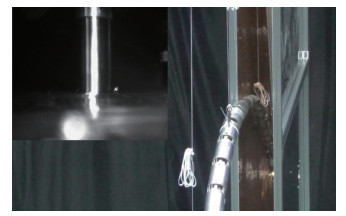图 15 吊丝配重系统下机械臂实物图 Fig.15 Physical diagram of the manipulator under the suspension system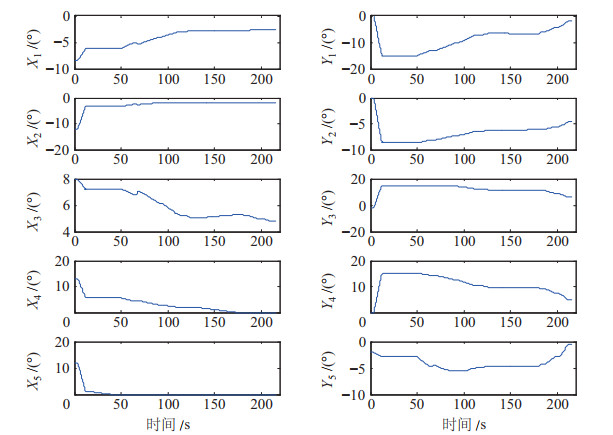图 16 关节角度曲线 Fig.16 The curve of joint angles
5 结论（Conclusion）

  刘天亮. 面向狭小空间作业的绳索驱动超冗余机械臂的研究[D]. 深圳: 哈尔滨工业大学, 2016. Liu T L. A study on the cable-driven and hyper redundant manipulator for the narrow space operations[D]. Shenzhen: Harbin Institute of Technology, 2016.  Hirzinger G, Brunner B, Dietrich J, et al. Sensor-based space robotics-ROTEX and its telerobotic features[J]. IEEE Transaction on Robotics and Automation, 1993, 9(5): 649-663. DOI:10.1109/70.258056  Oda M, DoiT. Teleoperation system of ETS-ⅤⅡ robot experiment satellite[C]//IEEE/RSJ International Conference on Intelligent Robots and Systems. Piscataway, USA: IEEE, 1997: 1644-1650.  Ma O, Wang J, Misra S, et al. On the validation of SPDM task verification facility[J]. Journal of Robotic Systems, 2004, 21(5): 219-235. DOI:10.1002/rob.20011  Artigas J, Balachandran R, de Stefano M, et al. Teleoperation for on-orbit servicing missions through the ASTRA geostationary satellite[C]//IEEE Aerospace Conference. Piscataway, USA: IEEE, 2016. DOI: 10.1109/AERO.2016.7500785.  王学谦, 徐文福, 梁斌, 等. 空间机器人遥操作系统设计及研制[J]. 哈尔滨工业大学学报, 2010, 42(3): 337-342. Wang X Q, Xu W F, Liang B, et al. Design and development of teleoperation system for space robot[J]. Journal of Harbin Institute of Technology, 2010, 42(3): 337-342.  张涛, 陈章, 王学谦, 等. 空间机器人遥操作关键技术综述与展望[J]. 空间控制技术与应用, 2014, 40(6): 1-9, 30. Zhang T, Chen Z, Wang X Q, et al. Overview and prospect of key technologies of teleoperation of space robot[J]. Aerospace Control and Application, 2014, 40(6): 1-9, 30. DOI:10.3969/j.issn.1674-1579.2014.06.001  Martín-Barrio A, Roldán J J, Terrile S, et al. Application of immersive technologies and natural language to hyper-redundant robot teleoperation[J]. Virtual Reality, 2020, 24(3): 541-555. DOI:10.1007/s10055-019-00414-9  Zhu C C, Song A G. Design of a 3-DOF parallel handcontroller[J]. Journal of Sensors, 2017. DOI:10.1155/2017/8162512  李家霖, 杨洋, 杨铁, 等. 外骨骼式遥操作主手设计及主从异构映射算法研究[J]. 机器人, 2020, 42(6): 651-660. Li J L, Yang Y, Yang T, et al. Design of exoskeletal teleoperation master device and research on master-slave isomerism mapping algorithm[J]. Robot, 2020, 42(6): 651-660.  Singh J, Srinivasan A R, Neumann G, et al. Haptic-guided teleoperation of a 7-DoF collaborative robot arm with an identical twin master[J]. IEEE Transactions on Haptics, 2020, 13(1): 246-252. DOI:10.1109/TOH.2020.2971485  Qin L, Huang F, Chen Z, et al. Teleoperation control design with virtual force feedback for the cable-driven hyper-redundant continuum manipulator[J]. Applied Sciences, 2020, 10(22). DOI:10.3390/app10228031  Wang Q Y, Jiao W H, Yu R, et al. Modeling of human welders' operations in virtual reality human-robot interaction[J]. IEEE Robotics and Automation Letters, 2019, 4(3): 2958-2964. DOI:10.1109/LRA.2019.2921928  Wang H Y, Mecham R, Zhang B. A method targeting repair in space: Tele-operating a collaborative robot with virtual reality[C]//IEEE 7th Annual International Conference on CYBER Technology in Automation, Control, and Intelligent Systems. Piscataway, USA: IEEE, 2017: 1068-1071.  Liu T L, Xu W F, Yang T W, et al. A hybrid active and passive cable-driven segmented redundant manipulator: Design, kinematics, and planning[J]. IEEE/ASME Transactions on Mechatronics, 2021, 26(2): 930-942.  Mu Z G, Yuan H, Xu W F, et al. A segmented geometry method for kinematics and configuration planning of spatial hyper-redundant manipulators[J]. IEEE Transactions on Systems, Man, and Cybernetics: Systems, 2020, 50(5): 1746-1756.